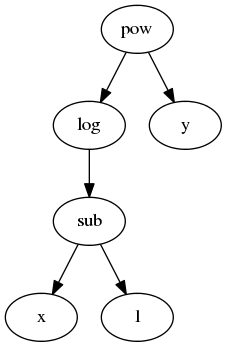# Expression Design¶

At its core Blaze expresses analytics queries symbolicly. It represents these queries as an abstract expression tree. This tree dictates user interaction, optimizations, and is eventually walked to translate into other computational systems. Deep knowledge of abstact expressions is not necessary to use Blaze; it is essential to develop in it.Consider the following example:

```>>> from blaze import symbol, log
>>> x = symbol('x', 'int64')
>>> y = symbol('y', 'float32')
>>> z = log(x - 1)**y
```

We represent the mathematical expression `log(x - 1)**y` as a tree where every operator (e.g. `log`, `pow`) is a node whose children are its arguments. All Blaze expressions and indeed all expressions in any programming language can be represnted this way. Blaze encodes this tree as a data structure where every node is an object with type corresponding to its operation. For example there exists the following classes

```class pow(Expr):
...
class sub(Expr):
...
class log(Expr):
...
```

And our expression, written explicitly as Blaze sees it, would look like the following:

```>>> from blaze.expr import Pow, Sub, log, symbol
>>> z = Pow(log(Sub(symbol('x', 'int64'), 1)),
...         symbol('y', 'float32'))
>>> z
(log(x - 1)) ** y
```

## Common Expression Attributes¶

There are a few important attributes and methods to investigate a Blaze expression.

• `__class__`: The type of a node corresponds to its operation:

```type(z) == pow
```
• `dshape`: The shape and type of the data for this expression. This is often more important that the actual type of the node:

```.. code-block:: python
```
```>>> s = symbol('s', 'var * float64')
>>> s.dshape
dshape("var * float64")
>>> s.mean().dshape
dshape("float64")
```
• `._args`: All children of a node, including parameters. Args may include both Blaze expressions and other variables like strings:

```z._args == (log(x - 1), y)
x._args == ('x', 'int64')
```
• `._hashargs`: The arguments in a form that is hashable. This is only different from `._args` when the arguments contain things that are not normally hashable with the `hash` builtin function.

• `._inputs`: All children of a node, excluding parameters. All inputs are Blaze expressions.:

```z._inputs == (log(x - 1), y)
x._inputs == ()
```
• `._leaves()`: The symbols at the bottom of the expression tree:

```z._leaves() == (x, y)
x._leaves() == (x,)
```

By recursively traversing either `._args` or `._inputs` you may inspect or transform either all information pertaining to the tree or just the expressions.

To clear up confusion between `._args` from `._inputs` consider the following tabular example with sort. `._inputs` contains only other Blaze expressions while `._args` also contains parameters like the string `'balance'`.

```>>> t = symbol('t', 'var * {name: string, balance: int}')
>>> expr = t.sort('balance', ascending=True)
>>> expr._args
(<`t` symbol; dshape='...'>, 'balance', True)
>>> expr._inputs
(<`t` symbol; dshape='...'>,)
```

Some convenience functions for common traversals already exist:

• `._subs`: replace nodes in the tree according to replacement dictionary:

```>>> z
(log(x - 1)) ** y
>>> z._subs({'x': 'a', 'y': 'b'})
(log(a - 1)) ** b
```
• `._subterms`, a traversal along `._inputs`:

```>>> list(z._subterms())
[(log(x - 1)) ** y, log(x - 1), x - 1, <`x` symbol; dshape=...>, <`y` symbol; dshape=...>]
```
• `._traverse`, a traversal along `._args`:

```>>> list(z._traverse())
[(log(x - 1)) ** y,
log(x - 1),
x - 1,
<`x` symbol; dshape=...>,
'x',
dshape("int64"),
0,
1,
<`y` symbol; dshape=...>,
'y',
dshape("float32"),
0]
```